Modeling Population Growth with Sequences

Lesson Objectives:

1. The mathematical modeling process

2. Definition of a sequence

3. Using Maple to plot sequences

Mathematical Modeling - The Process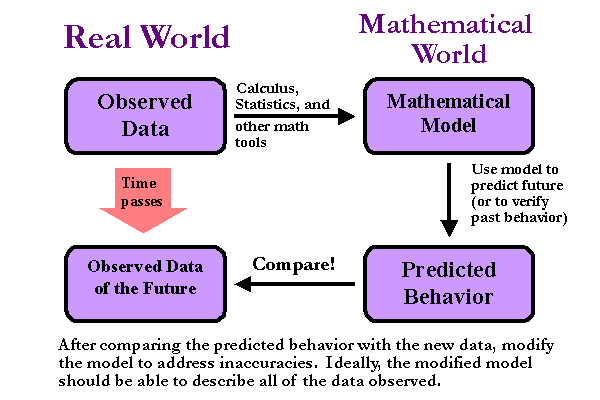Example. The world's population recently surpassed 6 billion...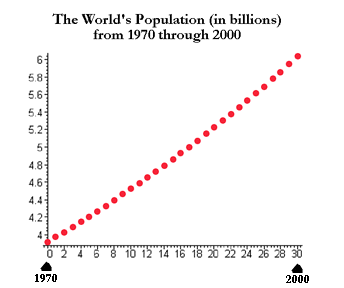(See http://metalab.unc.edu/lunarbin/worldpop for more recent data.)

To predict the world's population at a later time, construct a mathematical model...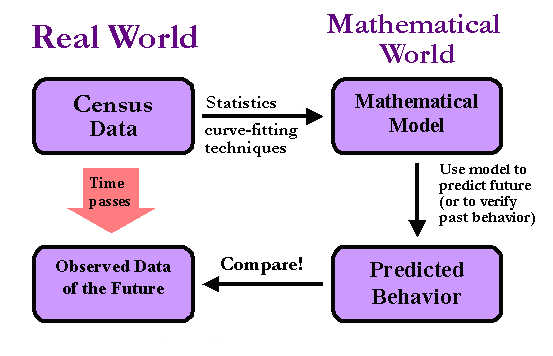Observe: Scientific data is usually taken at equally spaced increments of time (e.g., hourly, monthly, or annually), and the order in which the measurements are taken matters.

Hence, in analyzing data, we will be working with ordered lists of real numbers. Mathematicians call orders sets of real numbers sequences.

Sequences...

Definition.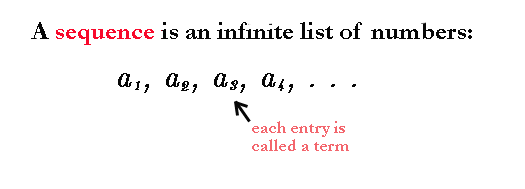A better way to say this is:

Definition.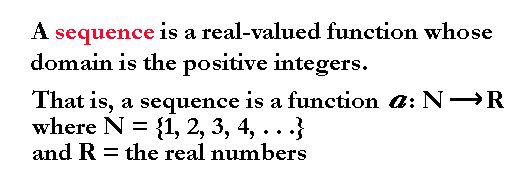Notation: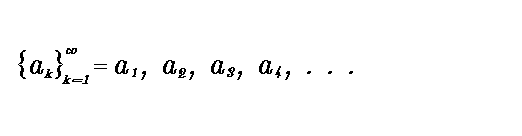It's worth mentioning that not all sequences start at i = 1. To cite an example, suppose that the ith term of a sequence {Pi} is defined by: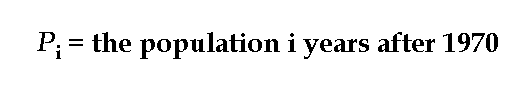then P0 represents the population in 1970, and P30 is the population in the year 2000.

Visualizing sequences...

The plot of a sequence, say {Pi}, is much like the plots of functions you have seen in the past (e.g., those commonly encountered in pre-calculus), but you won't get a smooth curve. The domain of a sequence is the set of positive integers. Hence the plot of a sequence {Pi} consists of bunch of "dots", with each dot placed over a positive integer i -- at a height determined by the value of Pi.

Example.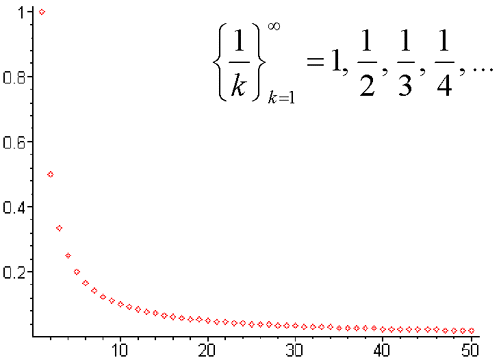This sequence can also be visualized as a subset of the curve defined by the function f(x) = 1/x defined over all positive real numbers: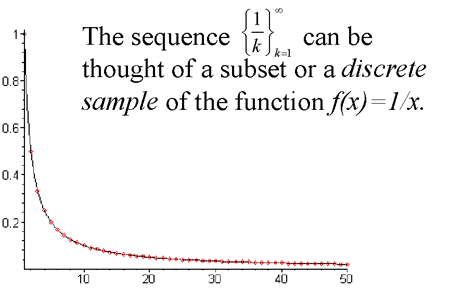Like any function, sequences can be described in several different ways:

A sequence can be described graphically: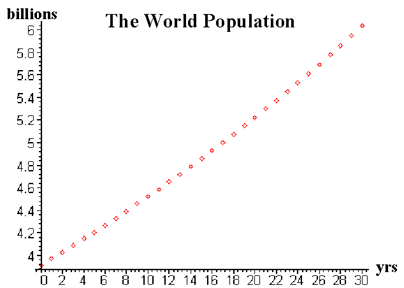A sequence can be described numerically:

 Year World Population 1970 3.912 billion 1971 3.969 billion 1972 4.027 billion 1973 4.086 billion 1974 4.145 billion 1975 4.205 billion 1976 4.266 billion 1977 4.329 billion . . . . . .

A sequence can be described symbolically: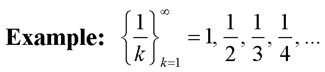The symbolic representation is nice because a formulaic description of the ith term of a sequence allows for the determination of any term of the sequence. In the case of a population sequence, this would mean that you could predict the population at any year simply by plugging in the appropriate value for i. Of course, in real life, such formulaic descriptions are hard (if not impossible) to find. In fact, often times mathematicians have to settle for numerical descriptions.

Sequences defined recursively. . .

In this class we will be interested, primarily, in sequences that are defined recursively.

Definition.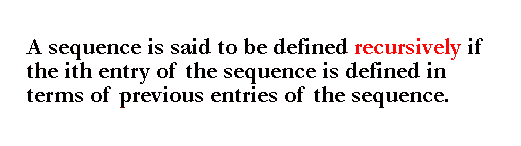Example.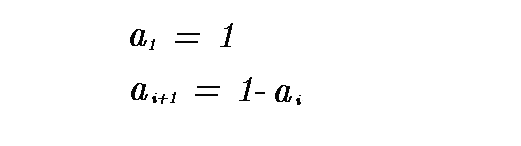This recursive description yields the sequence {1, 0, 1, 0, 1, 0, . . . }.

Note that in this example, we needed to know that the first term was 1 in order to get the second term: a2 = 1 - a1 = 0. Then, of course, we needed the second term to get the third, and the third term to get the forth, etc. Without the information about the first term we would not be able to determine the rest, and the definition would be faulty.

A recursive definition of a sequence requires two parts:

1. A starting point

and

2. A symbolic description of the ith term (or i+1st term) that depends on previous terms of the sequence

 Back to the Math 108 Course Schedule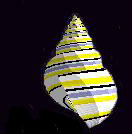Back to the Models of Life homepage Last modified: September 22, 2007